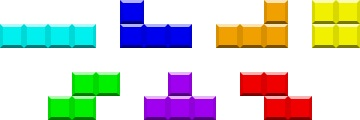# Creating Tetris Using Visual C++ Windows Form. Part one.

Tetris is an old and simple game of the puzzle matching kind. This game is a good example of creating a game application for every beginner developer. This tutorial is divided into two parts: one part is about game logic and the second one is about creating a Windows Forms Application that will interact with the user(player). When you develop any kind of the application, you should always keep in mind that you need to divide application's data and app's interface. The traditional Tetris game has 7 different types of the shapes:Let's call thess types in the program in this way: line, square, rightL, leftL, pyramide, leftZ, rightZ. To use these notation, we can create an enum class `ShapeType`:
`#pragma onceenum class ShapeType{	line, square, rightL, leftL, pyramide, leftZ, rightZ};`
Now we can start to implement class `Shape` that will produce different types of the shapes. The shape class will have two data members:
`array<Boolean,2>^	cells; // 2D Array for shapeShapeType	curType; // type of shape`
The constructor of the class will simply initialize the 2D array:
`Shape(){     cells = gcnew array<Boolean,2> (4,4);}`
As you can see, the Shape in this class is represented by boolean values.So,different types of shapes are different boolean arrays. For different shape we will need the different values for 2D array. This action is done in `void    NewShape(ShapeType type)` function. Let's take a look on the different types of shapes produced by it. Initially we need to clear the shape array:
`ClearShape(cells);Int16 i;curType=type;`
It's done by a simple function:
`void	ClearShape(array<Boolean,2>^ c) 	{		for(Int16 i = 0;i != 4;++i)		    for(Int16 j = 0; j != 4; ++j)			c[i,j] = false;	}`
Now,let's see how different types of shapes can be produced:
`switch(type)	{		case ShapeType::line:				for(i = 0; i != 4;++i)				  cells[0,i] = true;		break; 		case ShapeType::square:		  		for(i=0; i!=2; ++i)		    for(Int16 j=0; j != 2;++j)			cells[i,j]=true;			break; 		case ShapeType::leftL:				for(i = 0; i != 3;++i)		    cells[0,i] = true;		cells[1,2]=true;		break; 		case ShapeType::rightL:				for(i = 0; i != 3; ++i)		    cells[0,i]=true;		cells[1,0]=true;		break; 		case ShapeType::pyramide:			for(i = 0; i != 3; ++i)			cells[1,i]=true;		cells[0,1]=true;		break; 		case ShapeType::leftZ:				cells[0,0]=true; 		cells[1,0]=true;		cells[1,1]=true; 		cells[2,1]=true;		break; 		case ShapeType::rightZ:				cells[0,1]=true;		cells[1,0]=true;		cells[1,1]=true; 		cells[2,0]=true;		break;	}`
This code could be understood in a very good way by drawing a 3x3 grid and placing dots in the cells with true values. The most difficult operation in the Tetris game for programmer is the rotation of the shape. Some of the shapes is really easy to rotate, but some can put you in a trouble. The rotation of the line can be done in two ways, according to it's current position:
`switch(curType){case ShapeType::line:{Int16 k;if(cells[0,0]==true){	ClearShape(cells);	for(k = 0; k != 4; ++k)		cells[k,1] = true;}else{	ClearShape(cells);	for(k = 0; k !=4 ; ++k)		cells[0,k]=true;}return;}`
The square type should not be rotated:
`case ShapeType::square: return;}`
All the other shapes can be rotated using an universal rotation function:
`array<Boolean,2>^ tempShape = gcnew array<Boolean,2>(4,4);ClearShape(tempShape);for(Int16 j=3-1 , c=0; j>=0 ; j-- , c++)	for(Int16 i=0; i<3; i++)		tempShape[c,i]=cells[i,j]; ClearShape(cells);for(Int16 f=0; f<3; f++)	for(Int16 d=0; d<3; d++)		cells[f,d]=tempShape[f,d];}`
These piece of code just changes the position of the boolean values. And it can be used for any types of cells. Now, the Shape logic of the tetris game is implemented. In the next article you will find the information about how to create a Windows Form Application and link it's interface to the create Shape class.